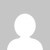# 18MATDIP31 ADDITIONAL MATHEMATICS – I Notes

[wptelegram-join-channel]

### 18MATDIP31 ADDITIONAL MATHEMATICS – I Notes

Her you can download the VTU CBCS 2018 Scheme notes, Study materials of 18MATDIP31 ADDITIONAL MATHEMATICS – I Notes.

Important topics Topics Covered

Complex Trigonometry, Vector Algebra: Scalar and vectors. Addition and subtraction and multiplication of vectors- Dot and Cross products, problems. Differential Calculus, Partial Differentiation: Euler’s theorem for homogeneous functions of two variables. Total derivatives -differentiation of composite function. Application to Jacobians of order two.

Vector Differentiation, Scalar and vector point functions. Gradient, Divergence, Curl and Laplacian (Definitions only). Solenoid and irrigational vector fields-Problems. Integral Calculus,  Double and triple integrals, problems.

Ordinary differential equations (ODE’s), s: Variable Separable methods, exact and linear differential equations of order one. Application to Newton’s law of cooling.

Full Notes

### 18MATDIP31 Additional Mathematics VTU Question Papers

18MATDIP31 Additional Mathematics VTU Question Papers

### 2 thoughts on “18MATDIP31 ADDITIONAL MATHEMATICS – I Notes”

1.1.